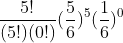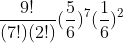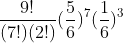## Thursday, 23 December 2010

### More "Almost Binomial" Distributions

In my recent post I illustrated the extension of the binomial to a Multinomial Distribution. In a similar way, the geometric distribution and the Pascal (aka the Negative Binomial) Distribution are very much like special cases of the binomial.

I will illustrate each with a simple probability example. In a limited version of the game of "greedy pig" you roll a die as many times as you wish each turn and you add the points on the top of the die to your score for that turn...but... if you roll a one, your turn ends and you lose all the points you have earned for that round. One might inquire, what is the probability that you could roll the die n times without rolling a one. Since the probability on each roll is the same, we could handle this using the binomial (or multinomial) distribution with n trials, p=5/6, and the number of successes also equal to n. For n=5 for example, we get (using the notation established in that blog)which simplifies to just (5/6)5 .

But a slightly different question might be, what is the probability that our first failure (rolling a one) would occur on the sixth roll. This is asking for the probability that the first five rolls succeed, and then the final roll is a failure. This is the general model for a geometric distribution. The reason it is called a geometric distribution is clear if you calculate the probability of the first failure happening on the first, second, etc rolls.

Roll...1....2.......3........4...

Prob...1/6..5/36...25/216 ..5^3/6^4..

notice that each probability is the previous probability multiplied by a constant ratio of 5/6. The terms for a geometric sequence (which must sum to one to be a probability distribution......check)

In general, if the probability of failure is q = 1-p.. then the probability of the first failure occurring on the nth trial is given by (p)n-1(q)

It often surprises students that the mean for such a distribution is 1/q where q is the probability of a failure. OK before I confuse someone.. the geometric distribution is sometimes described as the number of trials to the first success, so you may see the expected or mean value as 1/p. In any event, if the probability of an event happening (whether you call it success or failure) is p, the expected number of trials before it happens is 1/p.

Now if you are really clever you can figure out how to do the next problem without me, but let's walk through it anyway, (hey...it's MY blog).
Suppose instead, you could keep rolling until you had three rolls of one..... sort of "three strikes and you're out." Now what is the probability that the third strike comes on the tenth roll.
The idea of course, a collection of 9 rolls with 2 failures anywhere in the string, and then a third failure on the tenth roll. To get the probability of all the possible ways to get 7 successes and 2 failures in the first nine rolls is a straight binomial (multinomial) probability problem.We just multiply this by a failure on the tenth roll and we have the probability we seek. Since we have a couple of "failures" in that (1/6)^2, we might as well just up it to a three and be done. The final probability isIf you would like to experiment with these distributions, I came across a nice experimental applet hereThis experiment uses the trials to k successes instead of failures, and so p and q are switched here (and it seems I could only adjust these in .05 increments). This is a nice routine and you can simulate trials by clicking on the "step" button to see how many trials it took to get three successes.
This applet is part of a nice virtual laboratory created by Kyle Siegrist of the Department of Mathematical Sciences at the University of Alabama in Huntsville. There is lots of nice stuff. See the home page here.

When we deal with integer numbers of failures this is called a Pascal Distribution after Blaise Pascal. It can be extended to any real and is then called a Polya Distribution, after George Polya. This has application for events which are very rare, but related to each other, such as hurricanes. Both are special cases of the general Negative Binomial Distribution.